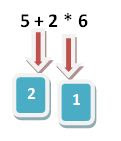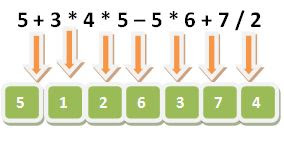# Explore hunderds of solved example topic wise.

## Operators

### Operators in C programming

Observe the given expression y = x + 5. In the above-given expression +, = are the operators and x, y, 5 are called operands/arguments.

#### Types of operator

According to the required argument the operator can be classified into 3 categories:

1. Unary operator: require only one argument. example(-5)
2. Binary operators: requires two arguments. example(5+8)
3. Ternary operator: require 3 arguments. example(?:)

According to the purpose operator are again classified into 8 types:

#### Arithmetic operator

+,-,*,/,%(mod) are called the arithmetic operator.

The +,-,* are the same as you learn in mathematical Arithmetic.

To more understand take an example.

`5 + 3 * 4`

In the above-given expression, which operator should be evaluated first? As in mathematics, you learn the rule of BODMAS, which tells first brackets should be evaluated, then divide, after that Multiply, addition, and subtraction.

In C there are more than 45 operators so BODMAS like rule doesn't work. For this purpose, the Rule of precedence is invented.

##### Rule of precedence

In C every operator enjoys the priority, The operator who enjoys the higher priority evaluates first and the operator of lower priority evaluates later.

##### Rule of association

The next rule for solving the expression is Rule of association.

This rule is used when in a single expression if there is more than one operator of the same priority occurs.

Rule of association tells that some operators are evaluated from left to right, and some others are evaluated from right to left. In the case of left to right associativity, the leftmost operator evaluates first and in the case of the right to left the rightmost operator evaluates first.

##### Precedence table of Arithmetic operator
OperatorAssociativity
* / %L to R
+ -L to R

Example-1:

In this example, the *(multiply) has a higher priority than (+)addition, so (*)multiply is evaluated before (+)addition.Example2:In this example, there are multiple operators of the same precedence, such as *,/ have the same precedence and +,- have the same precedence. Because *,/ have a higher priority than +,-. so *,/ are evaluated from left to right first and then +,- are evaluated from left to right.

#### Assignment operator

1. = operator is called the assignment operator.
2. It is used to move the value or expression result in a variable.
3. The assignment operator is evaluated from right to left order.

Example of assignment:

1. a = b = 5, In this example at first the 5 is assigned into b then b's value is moved to a.
2. a = b + 5, Assignment operator has the lowest precedence so all other operators are evaluated first and then the result is moved to the variable.
3. 5 = a: This is an error because in this example we are trying to move the value of the variable into constant and constant can't be changed. The same error will be prompted if you try the expression 6 + b = a.
##### Compound assignment
+=, -=, *=, /=, %= are called compound assignment operator. It combine the Arithmetic as well as assignment simultaneously.
Compound Assignment Equivalent Expression
a += 5 a = a + 5
a -= 5 a = a - 5
a *= 5 a = a * 5
a /= 5 a = a / 5
a %= 5 a = a % 5

#### Relational Operator

Relational operators are used to perform a logical test or create a condition. List of the logical operator is as given below...

Relational operator Meaning
> Greater Than
< Less Than
>= Greater Than or Equal to
<= Less Than or Equal to
== Equal to
!= Not equal
1. Every relational operator returns true or false.
2. In C any non-zero is treated as true and zero is treated as false.
3. In C if any expression evaluated true then the system returns 1 otherwise return 0.
4. Relational operators are evaluated from left to right.

Example1:

`printf("%d",5>2); //print 1`

Example2:

`printf("%d",5>2>1); //print 0//first 5>2 evaluated true and returns 1 and then result of this expression is compared with next 1, that is evaluated false.`

#### Logical Operator

1. &&(And) ||(Or) !(not) are the 3 logical operators.
2. &&(and), || (or) are used to combine two or more conditions.
3. Each condition can be true or false, so what will be the output of the combination made by logical operator &&(and), ||(or). This is decided by the truth table.
4. The truth table is the combination of conditions and their results as shown below...
C1 C2 C1 && C2 C1 || C2
F F F F
F T F T
T F F T
T T T T

Examples of Logical Operators

`int a = 5, b = 6, c;c = a > 2 && b > 3;printf("%d %d %d",a,b,c);//5 6 1`
`int a = 5 , b = 6, c;c = a > 2 || b < 8;printf("%d %d %d",a,b,c);//5 6 1`
```printf("%d",5 && 6);//1
//In c any non-zero is treated as true. because 5 and 6 are non-zero so both are true,so the result is true that is 1. ```
`int a = 5, b = 6, c;c = a > 2 || ++b;printf("%d %d %d",a,b,c);//5 6 1`

Logical operators are short circuit operator means OR(||) operator returns true as soon as if the first condition is true, AND(&&) operator returns false as soon as the first condition is evaluated false.

#### Not operator

Not operator just negates the result as the following truth table shown...

C !C
F T
T F

Example of not operator

```int a = 5, b;
b = !a > 2;
printf("%d %d",a,b);// 5 0
//NOT(!) have the greater precedence then (>). so first the !a is evaluated and results 0. After than 0>2 is evaluated that is false.
```
```int a = 5, b;
b = !(a > 2);
printf("%d %d",a,b);// 5 0 ```

#### Unary operators

Unary operator are those which only require one operand(argument) to operate. + , - , ++, --, typecast and sizeof are some unary

+5, -5 are the example of +,- unary operators. which represents +ve and -ve values.

Unary operators have high priority than binary operators, so unary operators are always evaluated first.

#### Increment / Decrement operator

++, -- are called increment and decrement operators.

Increment and decrement operators are available in two versions

1. Prefix
2. Postfix
##### Difference b/w prefix and postfix
Prefix Postfix
```int a = 5;
++a;
printf("%d",a); // 6
```
```int a = 5;
a++;
printf("%d",a); // 6
```
```int a = 5,b;
b = ++a;
printf("%d %d",a,b);// 6 6
```
```int a = 5,b;
b = a++;
printf("%d %d",a,b);//6 5
```
##### Example of increment and decrement operators
```int a = 3, b = 4,c;
c = ++a * ++b;
printf("%d %d %d",a,b,c); //4 5 20
```
```int a = 3,b = 4, c;
c = a++ * b++;
printf("%d %d %d",a,b,c); // 4 5 12
```
```int a = 3,b = 4, c;
c = ++a && b++;
printf("%d %d %d",a,b,c); //4 5 16
```
```int a = 5,b;
b = a++ * a++;
printf("%d %d",a,b); // 7 25
```
```int a = 5,b;
b = ++a * ++a;
printf("%d %d",a,b);
```
Above example, the output varies from compiler to compiler. on some compiler, the output is 7 42 as expected most of the time. but on the other hand, some compiler produces 7 49. This happens because on some compiler when the value of a is changed in one place then it affects all places of the same expression.

#### Comma operator

1. The comma operator is used to separate one or more variables and values.
2. Comma operator works from left to right but returns the rightmost value.
3. The comma operator is the last one in the priority table of the operator so it has the lowest priority in all operators.
```int a;
a = 5,6,7;
printf("%d",a); // 5
//because = operator enjoy the high priority than comma so it evalutes first and 5 gets stored in a.
```
```int a;
a = (5,6,7);
printf("%d",a); // 7
//Here the parenthisis is evaluted first and from multiple value right most value returns and gets stored in a so output is 7.```